# Calculating and visualizing Gimbal angles.

#### Mick West

Staff member
Thank you very much Mick! Although in the end the explanation proves that there is no other parameter that needs to be added to your beautiful tool, this one necessarily had to be done. Can you show me the code of this latest addition? I can better understand the behavior through.... numbers

This is the code (freshly cleaned and commented) that takes an Az value and converts it into CueAz (the angle of the Cue dot).
JavaScript:
``````// Take a frame number in the video (i.e. a time in 1/30ths)
// and return the angle formed by projecting the camera's Az/El vector
// onto the plane of the wings
function Frame2CueAz(frame) {

// get az for this frame (el is constant, in par.el)
// this comes from video data, shown on the graph as yellow
var az = Frame2Az(frame)

// get a unit vector in the direction of Az/El
// the Az=0 and El=0 is always along the -Z axis
// This can be thought of as a position on a unit sphere.
// El is relative to the horizontal plane, so AoA is irrelevant
// Az is relative to the jet's forward direction in the horizontal plane
var AzElHeading = EA2XYZ(par.el, az, 1)

// Create a Plane object, representing the wing plane
// (a "plane" is a flat 2D surface in 3D space, not an aeroplane)
// the plane in Hessian normal form, normal unit vector (jetUp)
// and a distance from the origin (0, as the origin is the ATFLIR camera, i.e. the jet)
var jetRoll = jetRollFromFrame(frame) // get jet roll angle from either video data or constant
var jetUp = new THREE.Vector3(0, 1, 0) // y=1 is the jet up unit vector with no rotation
jetUp.applyAxisAngle(V3(0,0,1),-radians(jetRoll)) // apply roll about Z axis (-Z is fwd, so -ve)
var wingPlane = new THREE.Plane(jetUp,0)

// now find the jet's forward vector, which will be in the wing plane
// same rotations as with the up vector
var jetForward = new THREE.Vector3(0, 0, -1)

// calculate the angle between the jet forward vector

// angleTo always returns a positive value, so we
// need to negate it unless cross product is in same direction as jet up
// the cross product will give a vector either up or down from the plane
// depending on if cueHeading is left or right of JetForward when looking down
// then use a dot product which returns positive if two vectors are in the same direction
var sign = cross.dot(jetUp)
if (sign < 0) cueAz = -cueAz

// The return value is plotted in cyan (light blue)
return cueAz
}``````

And the code that makes the arrows
JavaScript:
``````//  var rotationMatrix = new THREE.Matrix4().extractRotation(PodFrame.matrixWorld);
//  var jetUp = new THREE.Vector3(0, 1, 0).applyMatrix4(rotationMatrix).normalize();

// The rotations below are equivalent to the above,
// but I'm doing it explicitly like this
// to match the code in Frame2CueAz,
// so the graph uses the same code as the arrows
// except instead of using a unit sphere
// to get the large arrows in the display.
var jetUp = new THREE.Vector3(0, 1, 0)
var jetPlane = new THREE.Plane(jetUp,0) // plane in Hessian normal form, normal unit vector and a distance from the origin
// take the idealPos (the white dot) and project it onto the jetPlane
var cuePos=new THREE.Vector3;
jetPlane.projectPoint(idealPos,cuePos) // project idealPos onto jetPlane, return in cuePos
DebugArrowAB("Projected Cue",idealPos,cuePos,0x00ffff,false)
DebugArrowAB("Cue Az",V3(0,0,0),cuePos,0x00ffff,false)

var horizonPlane = new THREE.Plane(V3(0,1,0),0)
var azPos=new THREE.Vector3;
horizonPlane.projectPoint(idealPos,azPos) // the same as just setting y to 0
DebugArrowAB("Projected Az",idealPos,azPos,0xffff00,false)
DebugArrowAB("Az",V3(0,0,0),azPos,0xffff00,false)``````

You can also just view source with the tool itself. https://www.metabunk.org/gimbal/

#### Mick West

Staff member
I've deleted a large post by @dimebag2, as it's substantially off topic for this thread which is focussed on "calculating and visualizing gimbal angles", not on things like calculating probabilities of planes being in particular positions.

I do not do this lightly. Experience has show that allowing a wide variety of discussion topics in a complex subject results in massive thread drift, and the obscuration of the actual subject of the thread (see the 100 page 9/11 threads for example).

#### Leonardo Cuellar

##### Active Member
This is the code (freshly cleaned and commented) that takes an Az value and converts it into CueAz (the angle of the Cue dot).
Thanks again Mick. Observing the code and playing a bit on the tool, the cue dot is also a function of the elevation. And AOA and elevation are directly proportional. Very interesting.

#### Mick West

Staff member
Thanks again Mick. Observing the code and playing a bit on the tool, the cue dot is also a function of the elevation. And AOA and elevation are directly proportional. Very interesting.
AoA and elevation are only directly proportional when looking straight ahead.

#### Mick West

Staff member
I think we've got a bit of a terminology problem (which does not change the math).

AoA is not pitch unless we are in straight and level flight. AoA is relative to pitch. Here's a relatively clear explanation from Boeing's aero magazine: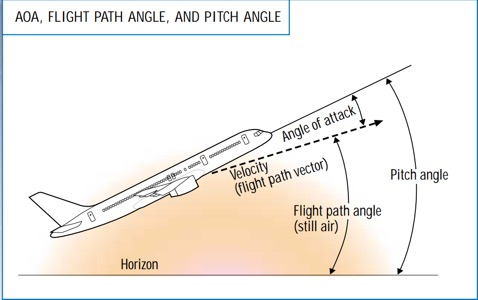Article:
Angle of attack (AOA) is the angle between the oncoming air or relative wind and a reference line on the airplane or wing. Sometimes, the reference line is a line connecting the leading edge and trailing edge at some average point on the wing. Most commercial jet airplanes use the fuselage centerline or longitudinal axis as the reference line. It makes no difference what the reference line is, as long as it is used consistently.

AOA is sometimes confused with pitch angle or flight path angle. Pitch angle (attitude) is the angle between the longitudinal axis (where the airplane is pointed) and the horizon. This angle is displayed on the attitude indicator or artificial horizon.

Flight path angle is defined in two different ways. To the aerodynamicist, it is the angle between the flight path vector (where the airplane is going) and the local atmosphere. To the flight crew, it is normally known as the angle between the flight path vector and the horizon, also known as the climb (or descent) angle. Airmass-referenced and inertial-referenced flight path angles are the same only in still air (i.e., when there is no wind or vertical air movement). For example, in a headwind or sinking air mass, the flight path angle relative to the ground will be less than that referenced to the air. On the newest commercial jet airplanes, this angle can be displayed on the primary flight display and is calculated referenced to the ground (the inertial flight path angle).

AOA is the difference between pitch angle and flight path angle when the flight path angle is referenced to the atmosphere. Because of the relationship of pitch angle, AOA, and flight path angle, an airplane can reach a very high AOA even with the nose below the horizon, if the flight path angle is a steep descent.

Now we ARE in level flight in Gimbal, but the angle I'm using to tilt the plane up and down is pitch, i.e. "the angle between the longitudinal axis (where the airplane is pointed) and the horizon"

This relates to the discussion by @markus about rotation order. What I do in the (rotate about the forward axis by the bank angle, and then about the sideways angle by the pitch angle) matches the definition of pitch, but I don't think it makes sense to describe that as AoA. Pitch is relative to the horizon. AoA is relative to airflow, so when banked it's not the same, and certainly not when climbing or descending. A banked turn could be thought of as "climbing" sideways through the air, so you can have a steep AoA with no pitch (for a bit)

So I think I will drop referring to the upward angle of the jet as the convenient "AoA", and refer to it just as pitch, and "jet pitch" to distinguish it from "Pod (ball) pitch".

#### Mick West

Staff member
Here's a cool little Attitude indicator simulator that shows how pitch is relative to the horizon
http://canvaslib.erau.edu/coa-flight/trainingaids/3dinstruments/attitude.html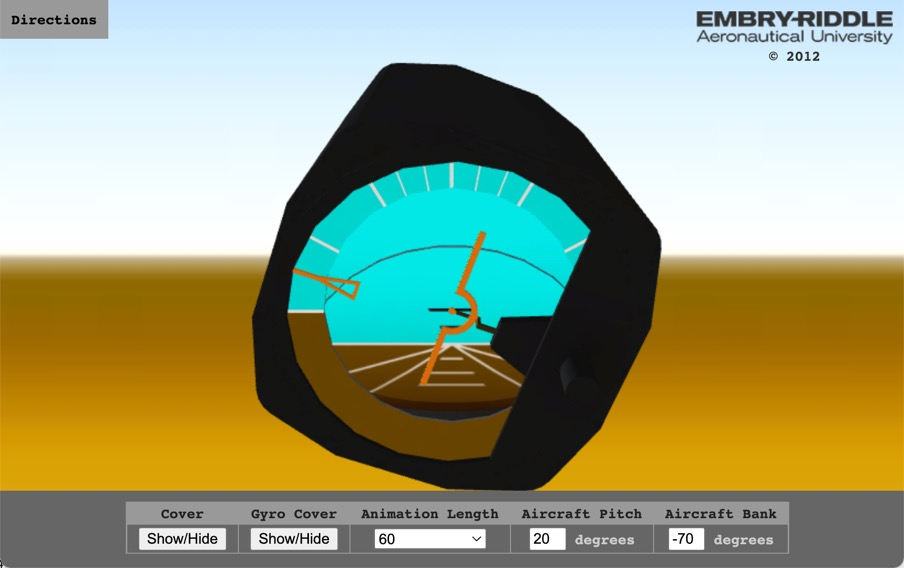Here I'm banked 70° left, pitch is 20° and it's shown as 20° above the horizon, which does not change with bank angle.

Rotate it around and hide the covers, you get a look at the inner workings.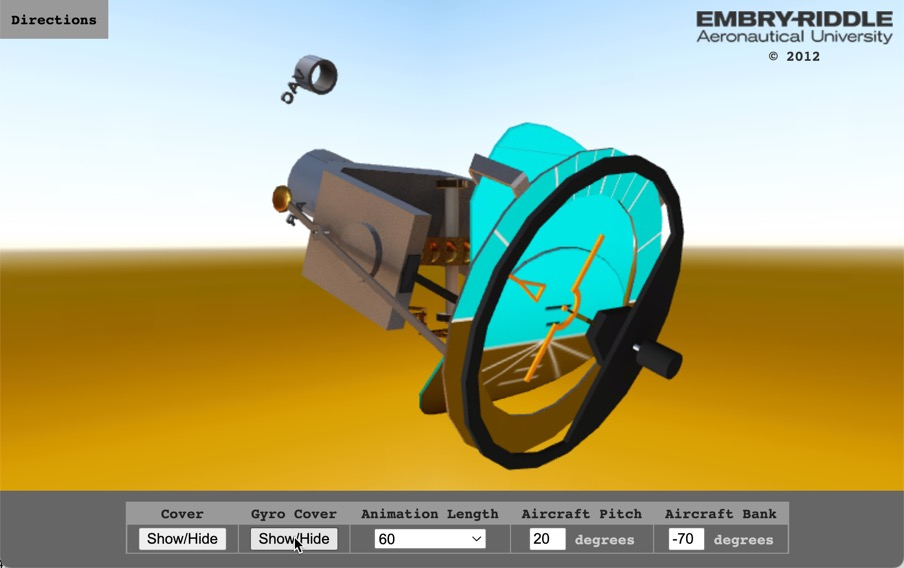#### Mick West

Staff member

A few updates to the sim. I've added the ability to drag the green target around - that represents the physical pointing direction, with a ring around it representing the 5° radius deviation allowed by internal gimballed mirrors.

There have also been a variety of performance improvements, allowing it to work on slower connections and a cleaner display on mobile.

Also, keyboard shortcuts are now displayed on the screen.
https://www.metabunk.org/gimbal/

#### markus

##### Active Member
Some very interesting developments this past weekWith hindsight, it's amusing that the resolution to this issue is pretty much the same as the resolution to the previous issue (compose two rotations in two different axes, and you end up rotating a bit in the remaining axis). Even having that fact in the immediate context this is not obvious.

I don't have much to add except to add some independent confirmation to some of the conclusions.

Before all this I was trying to estimate how the AoA would change as a function of bank angle, so I recorded AoA in DCS in two configurations, one with the jet laden with stores, another after jettisoning everything. I found this:

Heavy:
Code:
``````Bank | AoA
0°   | 6.7°
5°   | 6.7°
15°  | 6.8°
30°  | 7.5°
45°  | 8.9°``````

Light:
Heavy:
Code:
``````Bank | AoA
0°   | 5.6°
5°   | 5.6°
15°  | 5.8°
30°  | 6.4°
45°  | 7.8°``````

I found that in both cases these angles are modeled well in the region of interest by the following empirical relationship (bank in degrees):

AoA(bank) = AoA(0°) + bank^2.5 / 6160

This happens to nearly cancel the effect of projecting the AoA onto the vertical to obtain the pitch, so that in the end the pitch is almost constant throughout the first 31 seconds, which is consistent with the information we get from the cue dot. In the last few seconds with the rapid reduction in bank angle something weird happens. It might be there's a small departure from coordinated flight, but what happens is also described well by a sharp drop in the AoA.

So, assuming that the AoA at 0 bank angle would be 4.5°, we come to the following model: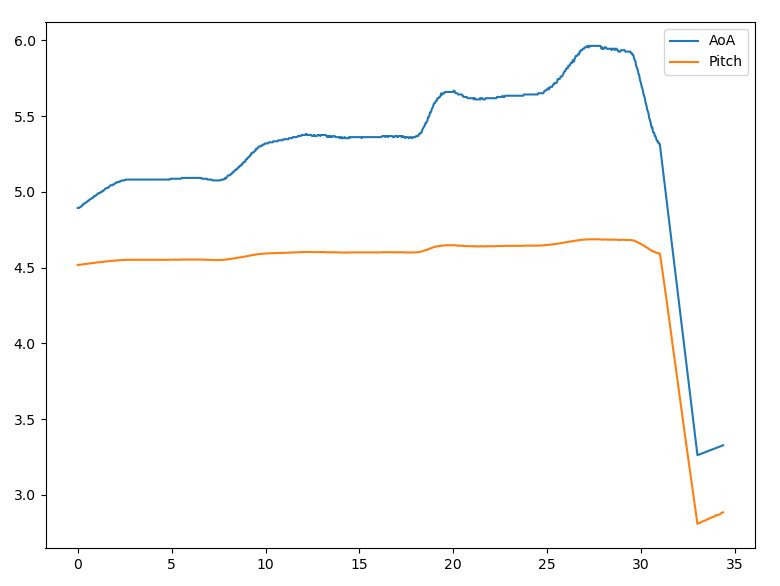which results in the following fit between predicted and actual pip angle:
: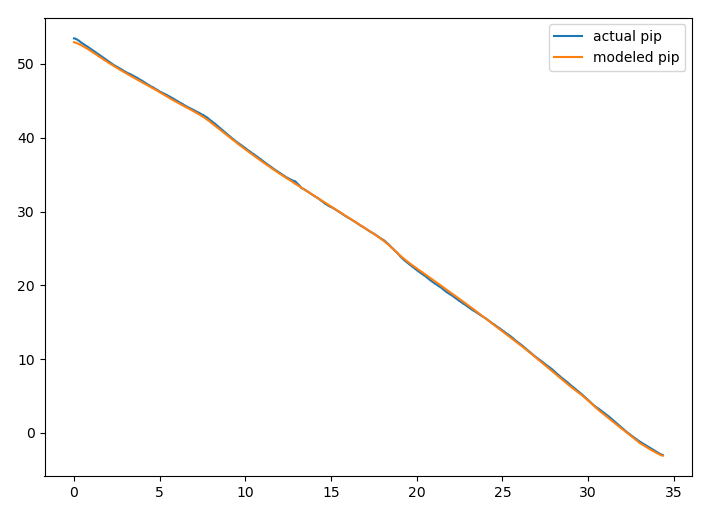(I'm not posting the whole code again because it's gotten quite messy, but since my product is the trajectory of the object in the frame of the jet, it's very simple to calculate: simply grab
`-np.arcsin(y / np.sqrt(x**2 + y**2))` after the bank rotation is applied).

There's bit of uncertainty left in the AoA even with the cue dot indication: we don't know if that indication has some lag, and the cue's trajectory on the screen is not a simple circular arc, which is strange (maybe it's indicating something else, or it's using some crummy approximation for the trigonometric functions). Still, its allowable range is narrowed and it's very nice to be confident that the azimuths really are with respect to the boresight. Great work!

#### Attachments

• aoa.csv
78.7 KB · Views: 112

#### Mick West

Staff member
There's bit of uncertainty left in the AoA even with the cue dot indication: we don't know if that indication has some lag, and the cue's trajectory on the screen is not a simple circular arc
I think it's simply clipping the radius, so it stays on-screen at the top.

Here's an interesting version I just made. Each frame is the brightest pixels in every 10th previous frame. This shows the path of the cue, and also some interesting "horizons" in the clouds as they move at different angles.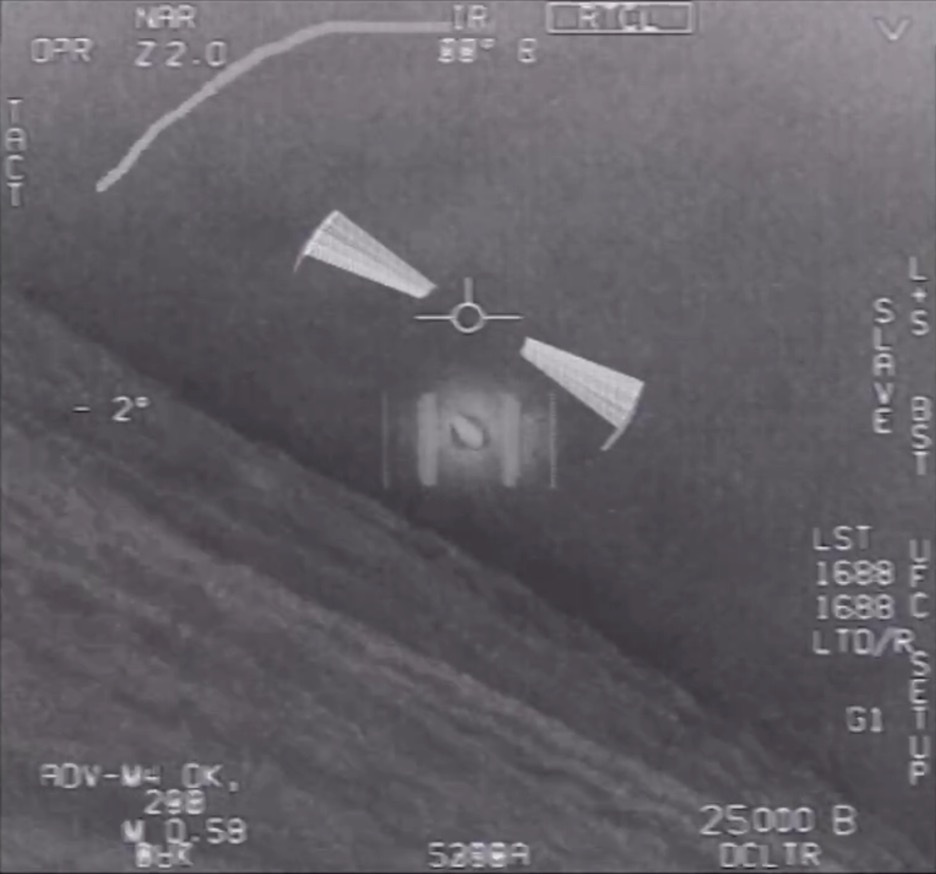#### Leonardo Cuellar

##### Active Member
Heavy:
Code:
``````Bank | AoA
0° | 6.7°
5° | 6.7°
15° | 6.8°
30° | 7.5°
45° | 8.9°``````
Light:
Heavy:
Code:
``````Bank | AoA
0° | 5.6°
5° | 5.6°
15° | 5.8°
30° | 6.4°
45° | 7.8°``````
I found that in both cases these angles are modeled well in the region of interest by the following empirical relationship (bank in degrees):

AoA(bank) = AoA(0°) + bank^2.5 / 6160
Excellent work!
The function that links the angle of attack to the bank angle should be the following (respecting the aerodynamic principles in which up to the stall angle of attack, this angle and the lift coefficient are directly proportional):
AoA = AoA0 * load factor
load factor = 1 / cos (bank angle)

#### dimebag2

##### Active MemberThat's cool. It makes the cloud relief much more apparent. And you finally found the Atlas Rocket!

#### Mick West

Staff member
Before all this I was trying to estimate how the AoA would change as a function of bank angle, so I recorded AoA in DCS in two configurations,Of course, they are the same in straight and level flight. But I wonder if this can be verified as being the same in a level banked turn (what Gimbal and GoFast are doing) in DCS? What instrument gives you the AoA? is this the same as the pitch angle on the artificial horizon?

To add to the naming confusion, "bank" and "roll" do not always refer to the same thing. I found a couple of useful sources. There's Section 3.3. and Appendix C of "Performance of the Jet Transport Airplane" by Trevor M. Young:
https://www.amazon.com/Performance-...&asin=B07ZJNT15C&revisionId=&format=4&depth=1

Section 3.3 (From the Kindle sample linked above) has a simple description.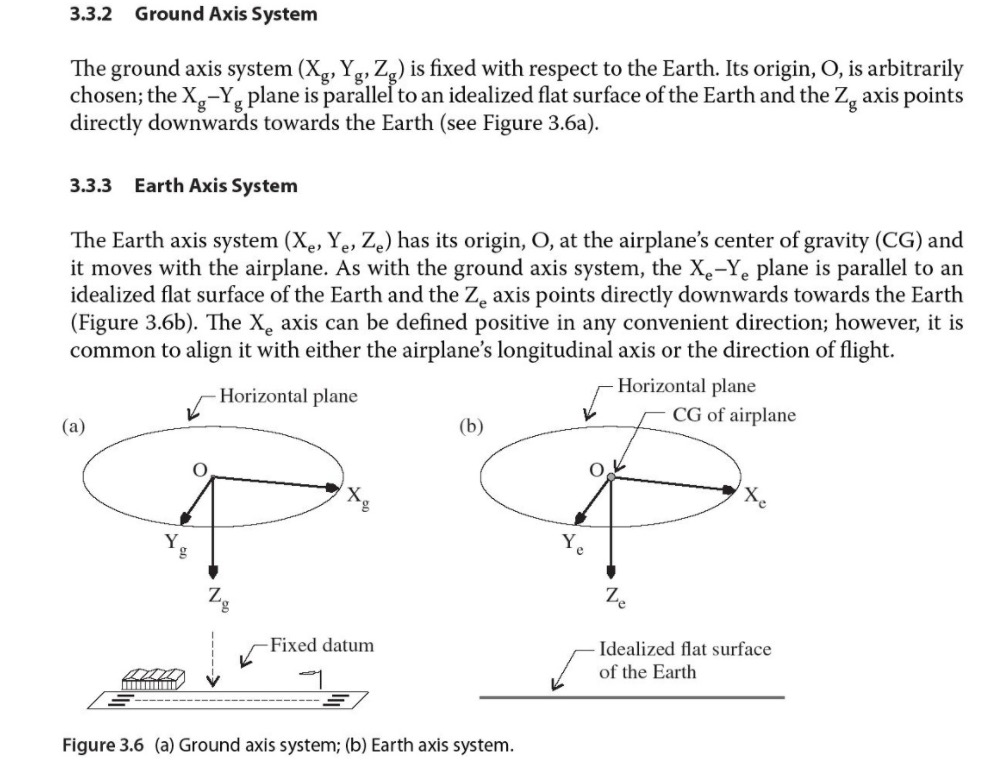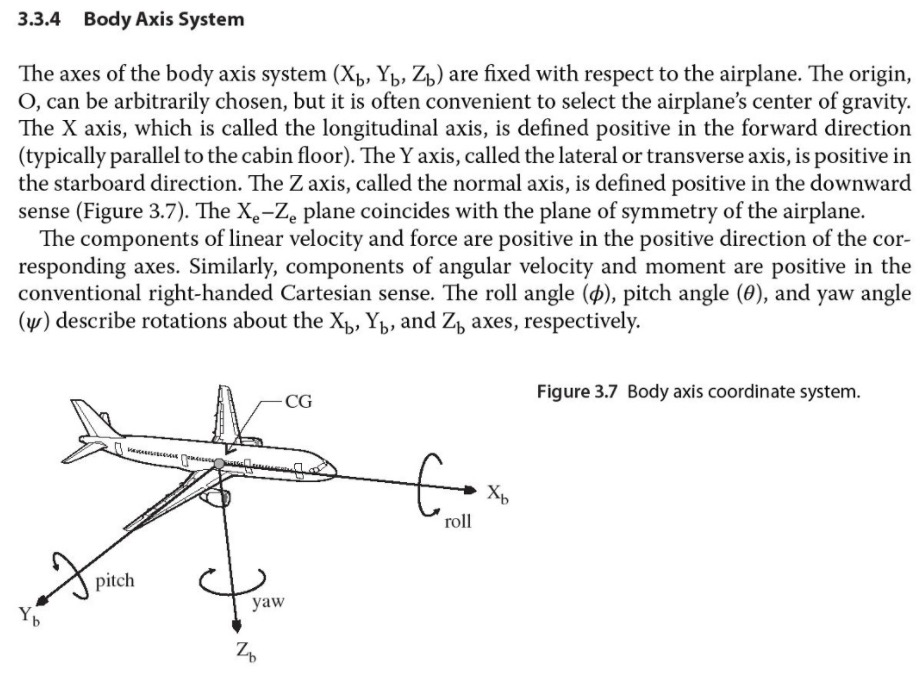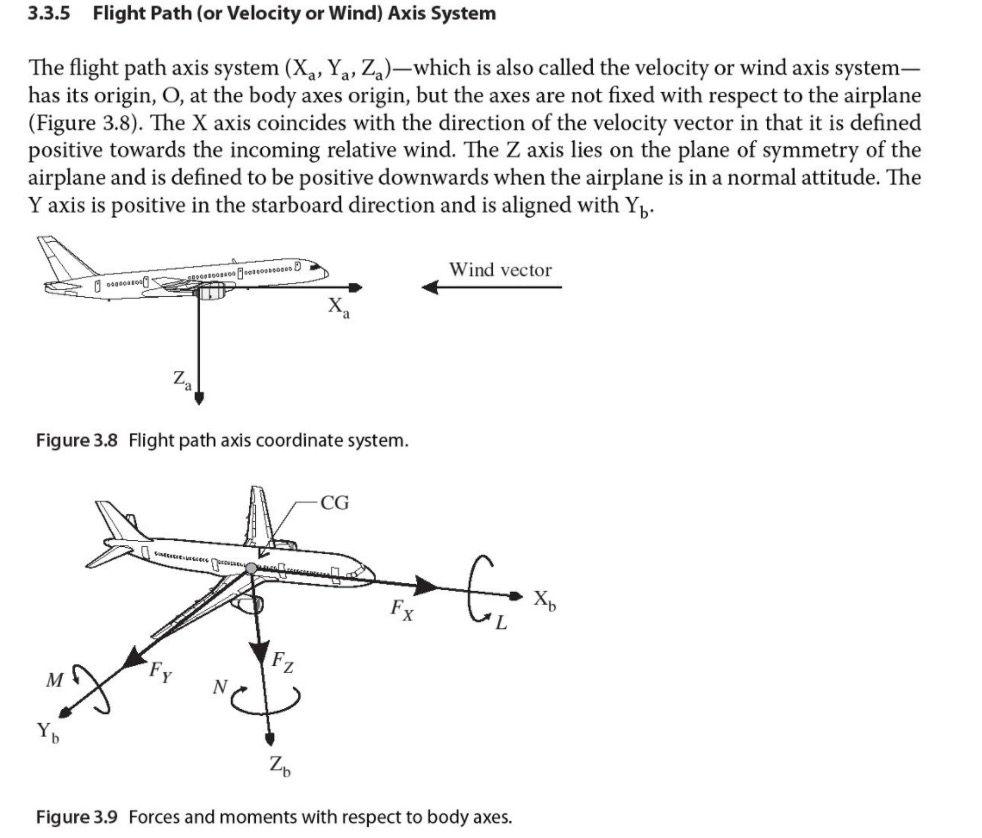The appendix describes the rotations in more detail, and in particular, it describes how "bank angle" is derived from "roll angle" and "pitch"
https://onlinelibrary.wiley.com/doi/pdf/10.1002/9781118534786.app3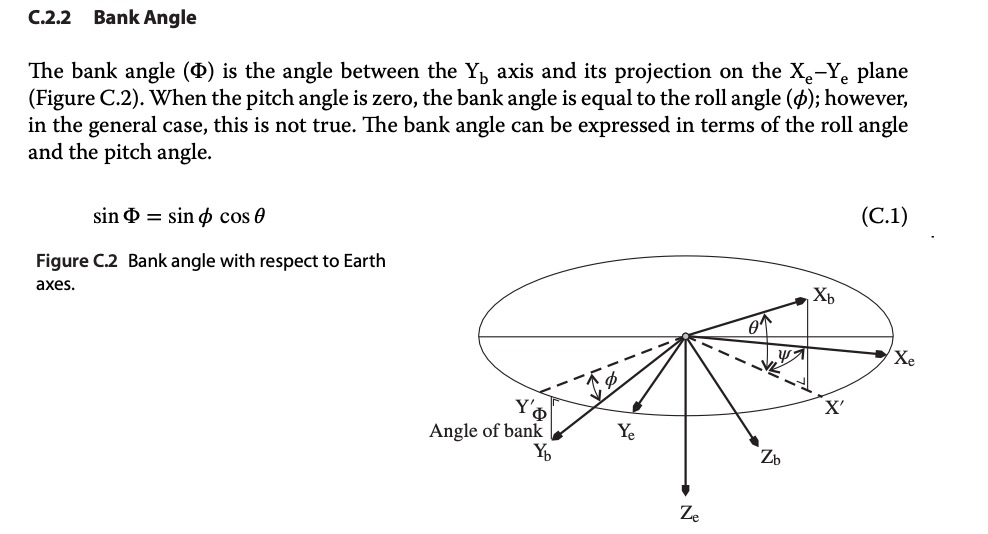sin(Bank) = sin (Roll) * cos(Pitch)
Bank = asin( sin (Roll) * cos(Pitch) )

This does not seem to be particularly significant for the pitch angles under question. At 6° pitch, and 30-degree roll, this gives:
asin(sin(30 degrees)*cos(6 degrees)) in degrees = 29.8 degrees - which is within the margin of error of the screen-scraped roll angle. Cos(6°) is 0.995

However, I'll try to refer to "roll" instead of "bank" - although they can currently be used interchangeably, it's best to be clear where possible.

#### Mick West

Staff member
The function that links the angle of attack to the bank angle should be the following (respecting the aerodynamic principles in which up to the stall angle of attack, this angle and the lift coefficient are directly proportional):
AoA = AoA0 * load factor
load factor = 1 / cos (bank angle)

It sounds like a lot, but in reality, it makes less than 1° of difference in the jet pitch. But I've added it to the sim, so now "Jet Pitch" is the pitch at 0°, which is scaled like:
JavaScript:
``````function jetPitchFromFrame(f=-1) {
if (f == -1) f=par.frame;
var jetPitch = par.jetPitch;
if (par.scaleJetPitch) {
var roll = jetRollFromFrame(f)
}
return jetPitch;
}``````

So I've reduced the default pitch to 3.6° for the best fit with the Cue line, which gives us a very nice fit of under 3° error, with consistent correction rolls. The adjusted pitch varies from 3.9° (at 22° roll) to 4.6° (at 38° roll)

The calculated Cue Az is the cyan line, regular Az is the yellow, the screen-scraped Cue az is the white line.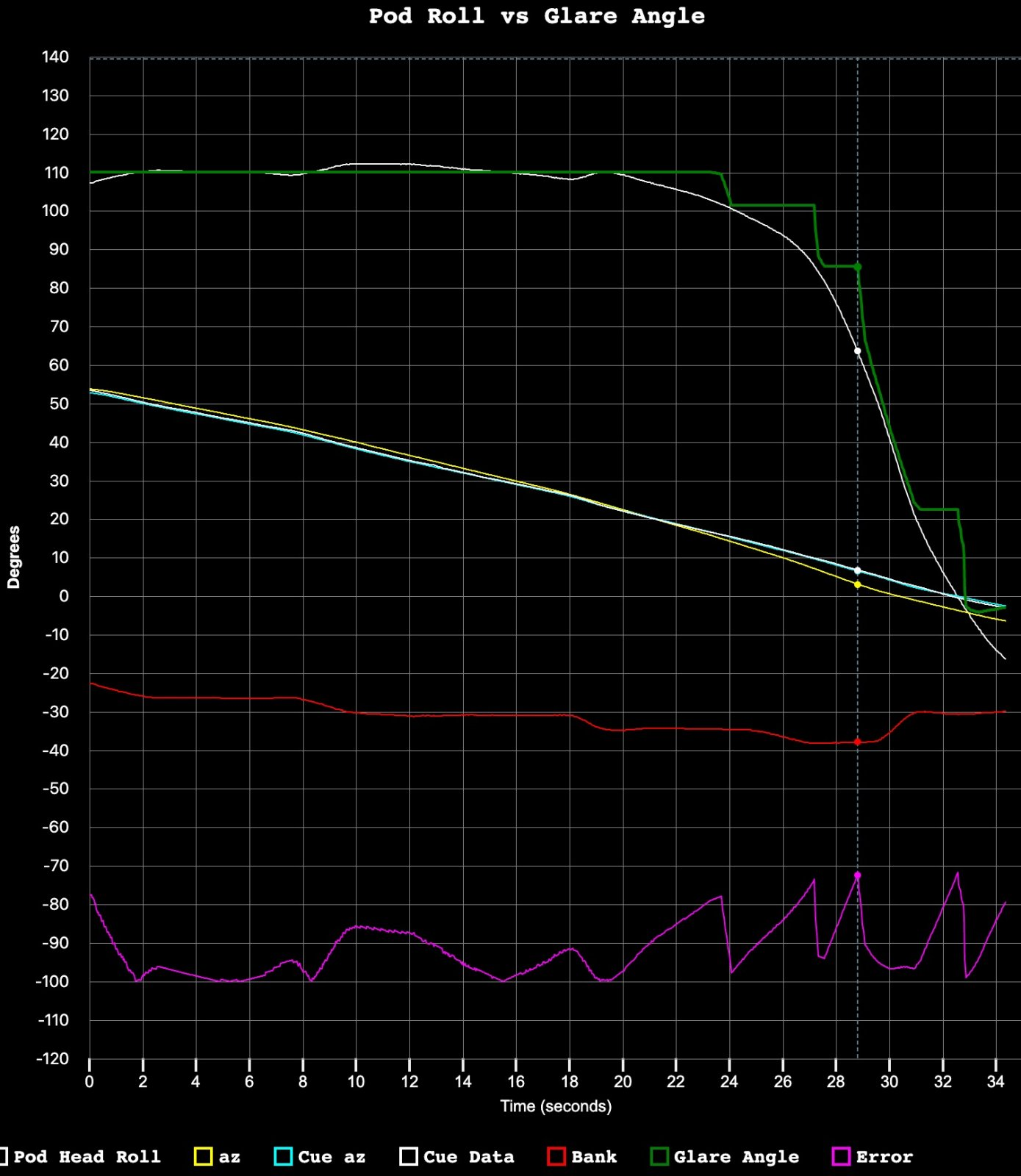The version with a fixed pitch, which is best at 4° to 5° (deviates in different places) , is also a pretty good fit. Here's a constant pitch of 4.6°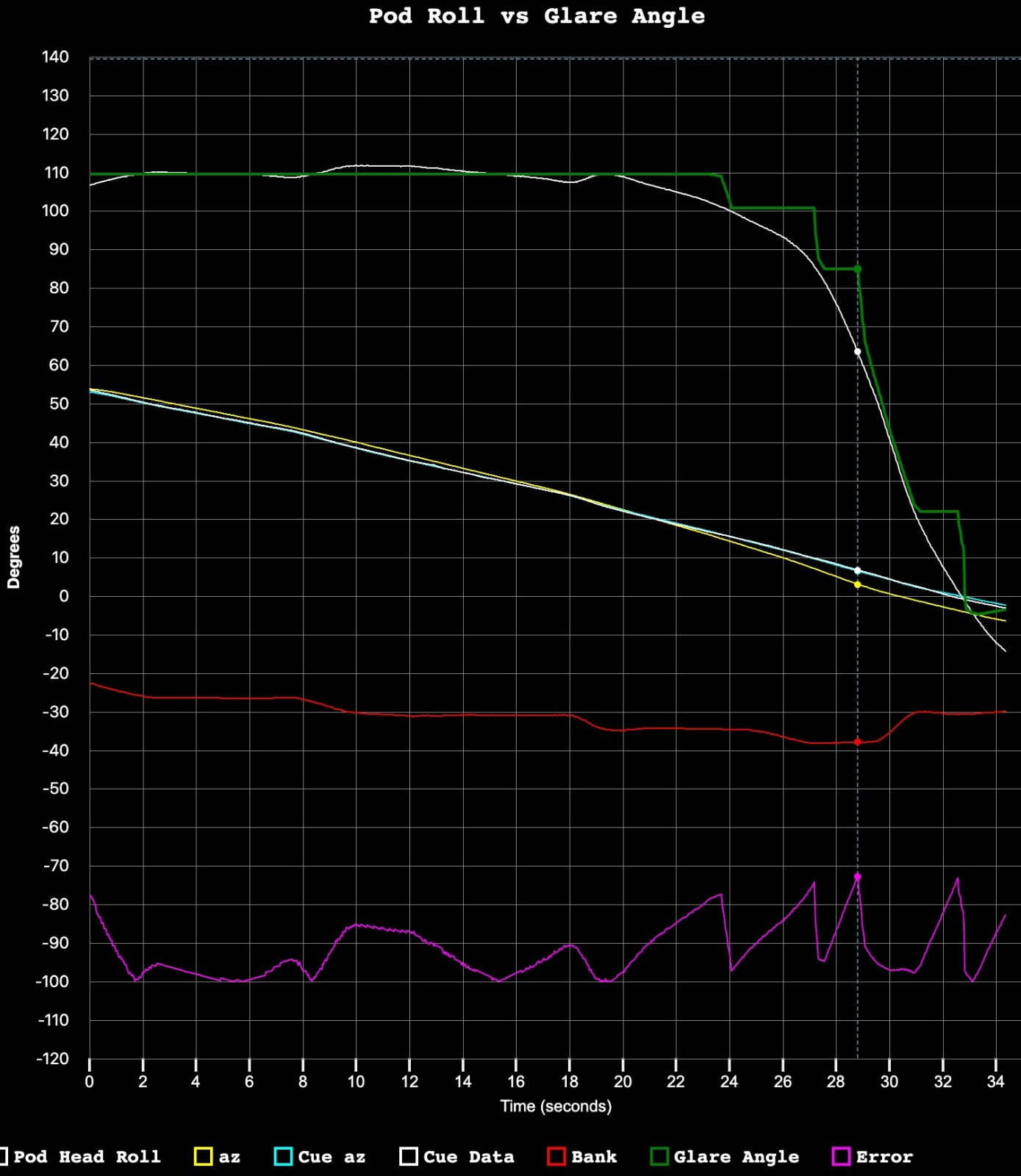The scaled pitch (i.e. nose up more to account for reduced lift in banks) is certainly better, but really just a minor refinement.

This value of 3.6° pitch (AoA, as in level flight), is lower than the values from DCS that @markus found. But it does not seem inconsistent with the values in the F/A-18 ops manual at 25,000 feet when optimizing for range.
https://info.publicintelligence.net/F18-EF-200.pdf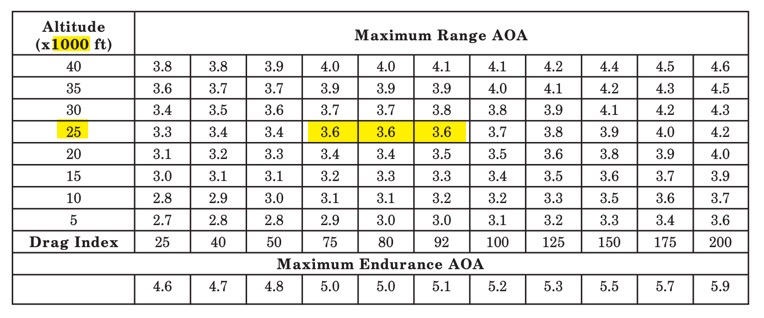#### Leonardo Cuellar

##### Active Member
This value of 3.6° pitch (AoA, as in level flight), is lower than the values from DCS that @markus found. But it does not seem inconsistent with the values in the F/A-18 ops manual at 25,000 feet when optimizing for range.
https://info.publicintelligence.net/F18-EF-200.pdfIn fact this table provides data for two different types of performance. Maximum range or maximum endurance. It would be necessary to know which of the two was applying the fighter to get back on the aircraft carrier. @markus data actually refer to a maximum endurance with minimum drag index, that is, with the fighter completely unloaded.
From the interpolation of the following graphs in the manual, it would seem that mach 0.58 is an optimal maximum endurance speed at 25000ft for a standard mission weight.

Last edited:

#### Leonardo Cuellar

##### Active Member
So, assuming that the AoA at 0 bank angle would be 4.5°, we come to the following model:I don't know how to interpret this graph, where to get the pip congruent to the actual pip, the pitch should drop dramatically by 2 ° in 4 seconds without the flight parameters undergoing significant variations.

#### logicbear

##### New Member
I calculated the elevation based on the measured cue angle data. With the default 3.6 degree base jet pitch I got the following graph: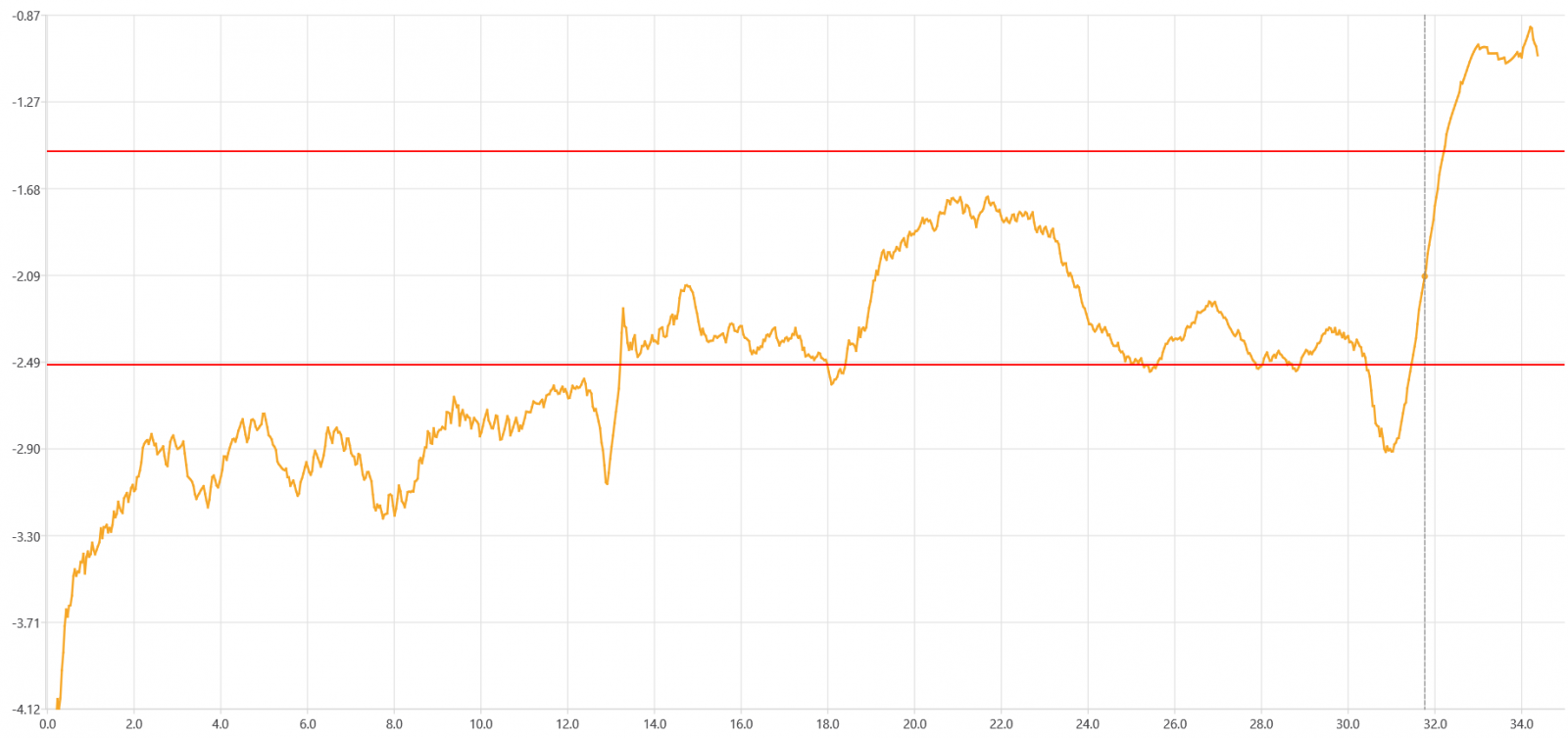The red lines indicate the range where the elevation should be, [-1.5,-2.5] to match the video. To verify the graph I used these elevation values to calculate the cue error, the difference between the calculated and measured cue heading angles, and I got errors under 0.03. If I change the base pitch it moves the whole graph up or down, can't get it all to stay in range, so it seems like there's something wrong here. Maybe the cue data is a bit off, maybe the pitch is a bit off, maybe the azimuth is a bit off, maybe the formula for calculating the cue heading/elevation is a bit off, maybe a combination of all that and more ?

I ported the Gimbal sim code to C++ and added a QML GUI. Here's the code I used for calculating the elevation:
C++:
``````double cue_data_to_elevation_for_frame(int frame, double jetPitch) {
// jetUp = Rx(pitch) Rz(-roll) up
// cueHeading = Rup(cue_data) Rx(pitch) Rz(-roll) fwd
// CH = P(jetUp) * AzEl <=> CH = AzEl + jetUp * d
// AzElx = cos(el) * sin(az)
// AzEly = sin(el)
// AzElz = -cos(el) * cos(az)
// CHx = cos(el) * sin(az) + jetUpx * d
// CHy = sin(el) + jetUpy * d
// CHz = -cos(el) * cos(az) + jetUpz * d
// cos(el) = (-CHz + jetUpz * d) / cos(az)
// CHx = (-CHz + jetUpz * d) * tan(az) + jetUpx * d
// CHx = d * (jetUpz * tan(az) + jetUpx) - CHz * tan(az)
// d = (CHx + CHz * tan(az)) / (jetUpz * tan(az) + jetUpx)
// el = arcsin(CHy - jetUpy * d)

double jetRoll = jetRollFromFrame(frame);
auto jetUp = vec3d { 0, 1, 0 }
.rotate(vec3d { 0, 0, 1 }, -radians(jetRoll))
.rotate(vec3d { 1, 0, 0 }, radians(jetPitch));

auto jetForward = vec3d { 0, 0, -1 }
.rotate(vec3d { 0, 0, 1 }, -radians(jetRoll))
.rotate(vec3d { 1, 0, 0 }, radians(jetPitch));

double cue_data = get_data(frame).Pip_Angle;

double d = (CH.x + CH.z * tan_az) / (jetUp.z * tan_az + jetUp.x);
double el = degrees(asin(CH.y - jetUp.y * d));

return el;
}``````
.

Last edited:

#### Mick West

Staff member
I ported the Gimbal sim code to C++
I'm not really clear what you are doing here. What's the code in comments there?

I'm using a constant elevation of 2°, and I get a great correlation between observed and projected cue (as discussed above)

#### Mick West

Staff member
To verify the graph I used these elevation values to calculate the cue error, the difference between the calculated and measured cue heading angles, and I got errors under 0.03.
What do you get if you just use el=2?

#### logicbear

##### New Member
I'm using a constant elevation of 2°, and I get a great correlation between observed and projected cue (as discussed above)
What do you get if you just use el=2?
With el=-2 it's a great correlation. Just like in your graphs the lines for the measured cue data (blue) and the calculated cue angle (red) overlap so well that you can barely tell them apart without zooming in. You can see in green the cue error for el=-2 magnified 100x. It's only off by +- half a degree but unless I'm doing something wrong that's enough to make most of the calculated elevation values fall outside of the required range.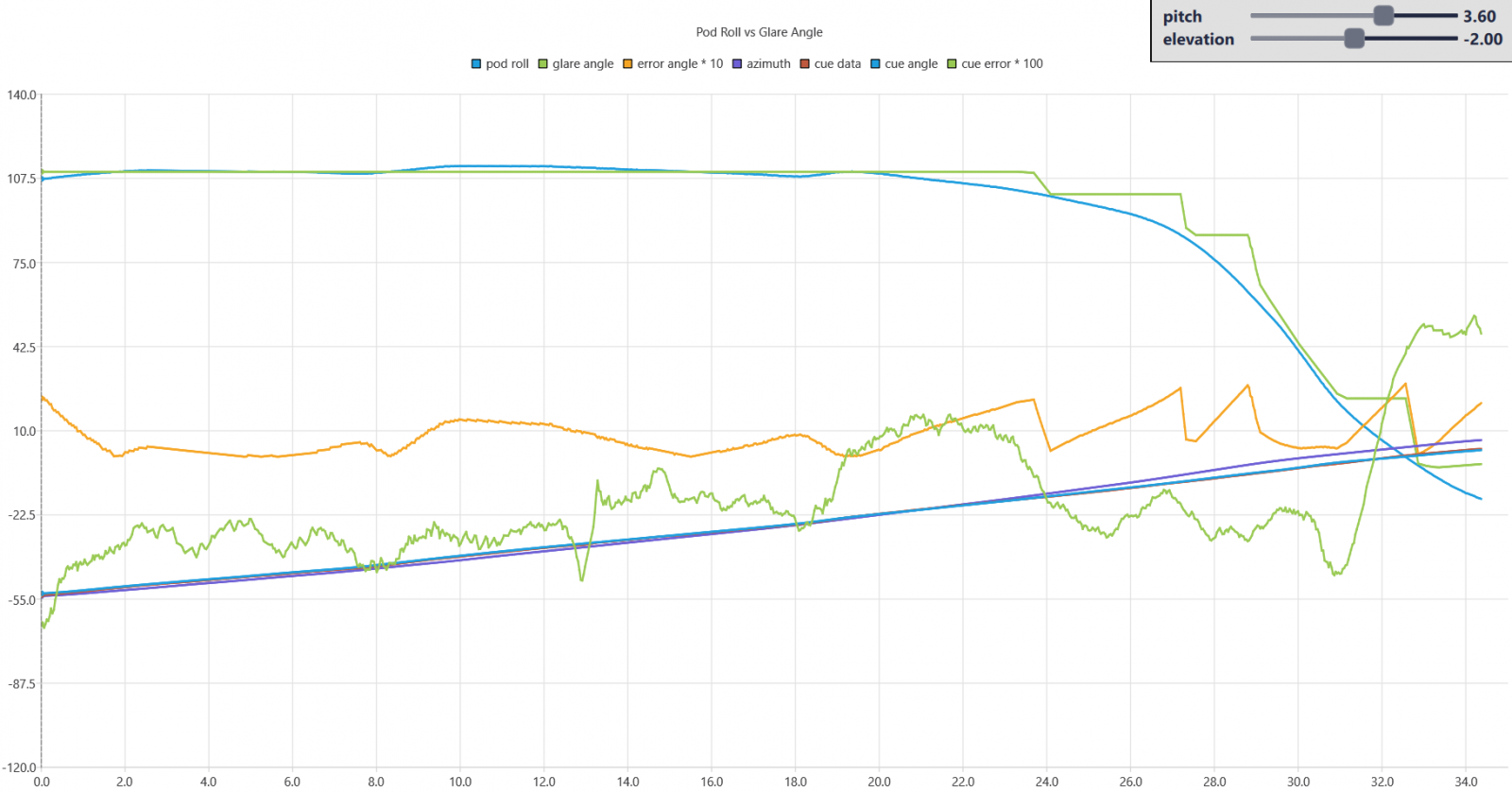The comments are mathematical notation that's there to briefly remind me how I derived elevation values:
The jetUp vector is the up axis multiplied first by a rotation around the z axis for the roll, then a rotation around the x axis for the pitch:
Code:
``jetUp = Rx(pitch) Rz(-roll) up``
The cueHeading vector (later referred to as CH) is the forward axis rotated first for roll, pitch, and then around the jetUp axis by cue_data, the measured cue angles from the CSV file:
Code:
``cueHeading = Rup(cue_data) Rx(pitch) Rz(-roll) fwd``
The EA2XYZ function derives an AzEl point from the elevation, azimuth values as follows:
Code:
``````AzElx = r * cos(el) * sin(az)
AzEly = r * sin(el)
AzElz = - r * cos(el) * cos(az)``````
The cue heading should be a projection of the AzEl point onto the plane defined by the jetUp normal. So there should exist some value 'd' which satisfies the condition:
Code:
``CH = AzEl + jetUp * d``
Substitute the AzEl components into the previous equation:
Code:
``````(1) CHx = r * cos(el) * sin(az) + jetUpx * d
(2) CHy = r * sin(el) + jetUpy * d
(3) CHz = - r * cos(el) * cos(az) + jetUpz * d``````
Derive r * cos(el) from (3):
Code:
``r * cos(el) = (-CHz + jetUpz * d) / cos(az)``
Substitute r * cos(el) into (1):
Code:
``CHx = (-CHz + jetUpz * d) * tan(az) + jetUpx * d``
Rearrange this and derive a formula for 'd':
Code:
``````CHx = d * (jetUpz * tan(az) + jetUpx) - CHz * tan(az)
d = (CHx + CHz * tan(az)) / (jetUpz * tan(az) + jetUpx)``````
Calculate AzEl using this value for 'd'
Code:
``AzEl = CH - jetUp * d``
Derive 'r' from (2), substitute that into (3), rearrange it and compute the elevation:
Code:
``````r = AzEly / sin(el)
AzElz = - AzEly / tan(el) * cos(az)
tan(el) = -AzEly * cos(az) / AzElz
el = atan2(-AzEly * cos(az), AzElz)``````
EDIT: I found a bug in my math above. Honestly I should've known that an error of 0.03 was way too large for it to be right. Now the cue error is under 10^-12. It doesn't change the elevation graph too much at that scale, the conclusion is still essentially the same, so I'm just posting the new code:
C++:
``````double cue_data_to_elevation_for_frame(int frame, double jetPitch) {
// jetUp = Rx(pitch) Rz(-roll) up
// cueHeading = Rup(cue_data) Rx(pitch) Rz(-roll) fwd
// CH = P(jetUp) * AzEl <=> CH = AzEl + jetUp * d
// AzElx = r * cos(el) * sin(az)
// AzEly = r * sin(el)
// AzElz = r * -cos(el) * cos(az)
// CHx = r * cos(el) * sin(az) + jetUpx * d
// CHy = r * sin(el) + jetUpy * d
// CHz = r * -cos(el) * cos(az) + jetUpz * d
// r * cos(el) = (-CHz + jetUpz * d) / cos(az)
// CHx = (-CHz + jetUpz * d) * tan(az) + jetUpx * d
// CHx = d * (jetUpz * tan(az) + jetUpx) - CHz * tan(az)
// d = (CHx + CHz * tan(az)) / (jetUpz * tan(az) + jetUpx)
// AzEl = CH - jetUp * d
// r = AzEly / sin(el)
// AzElz = - AzEly / tan(el) * cos(az)
// tan(el) = -AzEly * cos(az) / AzElz
// el = atan2(-AzEly * cos(az), AzElz)

double jetRoll = jetRollFromFrame(frame);
auto jetUp = vec3d { 0, 1, 0 }
.rotate(vec3d { 0, 0, 1 }, -radians(jetRoll))
.rotate(vec3d { 1, 0, 0 }, radians(jetPitch));

auto jetForward = vec3d { 0, 0, -1 }
.rotate(vec3d { 0, 0, 1 }, -radians(jetRoll))
.rotate(vec3d { 1, 0, 0 }, radians(jetPitch));

double cue_data = get_data(frame).Pip_Angle;

double d = (CH.x + CH.z * tan(az_rad)) / (jetUp.z * tan(az_rad) + jetUp.x);

vec3d AzEl = CH - jetUp * d;
double el = degrees(atan2(-AzEl.y * cos(az_rad), AzEl.z)) - 180;

return el;
}``````

Last edited:

#### logicbear

##### New Member
To further clarify what I was trying to achieve: The model for predicting the pod roll is already an incredibly good match for the measured glare angles, but perhaps there's still room for some tiny improvements. The model doesn't use the measured cue angle so I was wondering if that could be used to further refine the match. The cue data allows calculating the elevation from the pitch or vice versa, but based on my results so far it could be that it's just too imprecise to refine the match, that it just makes things worse unless we get better cue data ?

#### Mick West

Staff member
The cue data allows calculating the elevation from the pitch or vice versa, but based on my results so far it could be that it's just too imprecise to refine the match, that it just makes things worse unless we get better cue data ?
Yea, I don't think the cue data is particularly accurate. It's just a fuzzy dot on the screen that only provides the pilot a rough direction indicator. That was then extracted using feature tracking in Adobe After Effects.

The Az value isn't super accurate either. The ultimate arbiter of accuracy is getting the video to match - particularly the motion of the clouds.

Sitrec is basically layered on top of the original Gimbal sim (massively refactored) and differes in that:
1. Az uses "Az Editor" - a simple bezier curve, this isn't really needed JUST for cloud as the original "Markus Smoothed" is similar. But I was seeing if the clouds could be fixed by slight tweaks to the Az curve. However it really is needed to avoid excessive tweaking to the turn rate (see 3)
2. El starts at -2.01 and rises by 0.025°
3. The Turn rate is "Match Clouds", meaning the turn rate is calculated so the end direction of the camera matches the videos with a given cloud horizon motion (which is manually edited)
These are all very minor tweaks, like a degree or so over the entire video (0.025° for El) . But it shows how sensitive the whole system is - which is just a reflection of the long zoom.

One point is that I'm solving Turn Rate based on Az and Cloud motion. I could also calculate turn rate from bank angle and solve Az from turn rate.

And we're also ignoring wind here. I keep meaning to get around to adding it as a variable. That's going to bend a few curves.

#### logicbear

##### New Member
I've been working on some code to track the object and calculate its geometric properties. I've mostly used the video frames from the gimbal sim to make sure it lines up with the data. Recently I tried the higher resolution frames from the NYT's 75000_1_16_ufo-vid-2_wg_hd_synd.mp4 as well. Is that the highest quality version we have ? It would be helpful to make available a dataset with lossless quality images that are lined up with the rest of the data.

In order to be able to use the same binary threshold (0.2 * 255 currently) to find the object in both the white-hot / black-hot sections of the video, I did a histogram matching on a region of interest (tall rectangle between the tracking bars, down to the bottom of the image) between frames 369 (last white hot) and 371 (first black hot), then I applied the resulting matching function to the white-hot frames. The matching function I found is pretty close to y = 0.5 * ((255-x)/255)^3 * 255 - 12 , but I still wonder if the pointy edges of that are just noise and the gamma there is really more like the 2.2 that NTSC uses ?

I used image moments to find the center of mass and orientation. The algo doesn't work on frames that are full of interlacing or where the object moves behind the track bars, and there's a couple degrees of drift in the orientation which I think might be an artifact of the object changing shape, but it still matches the model quite well: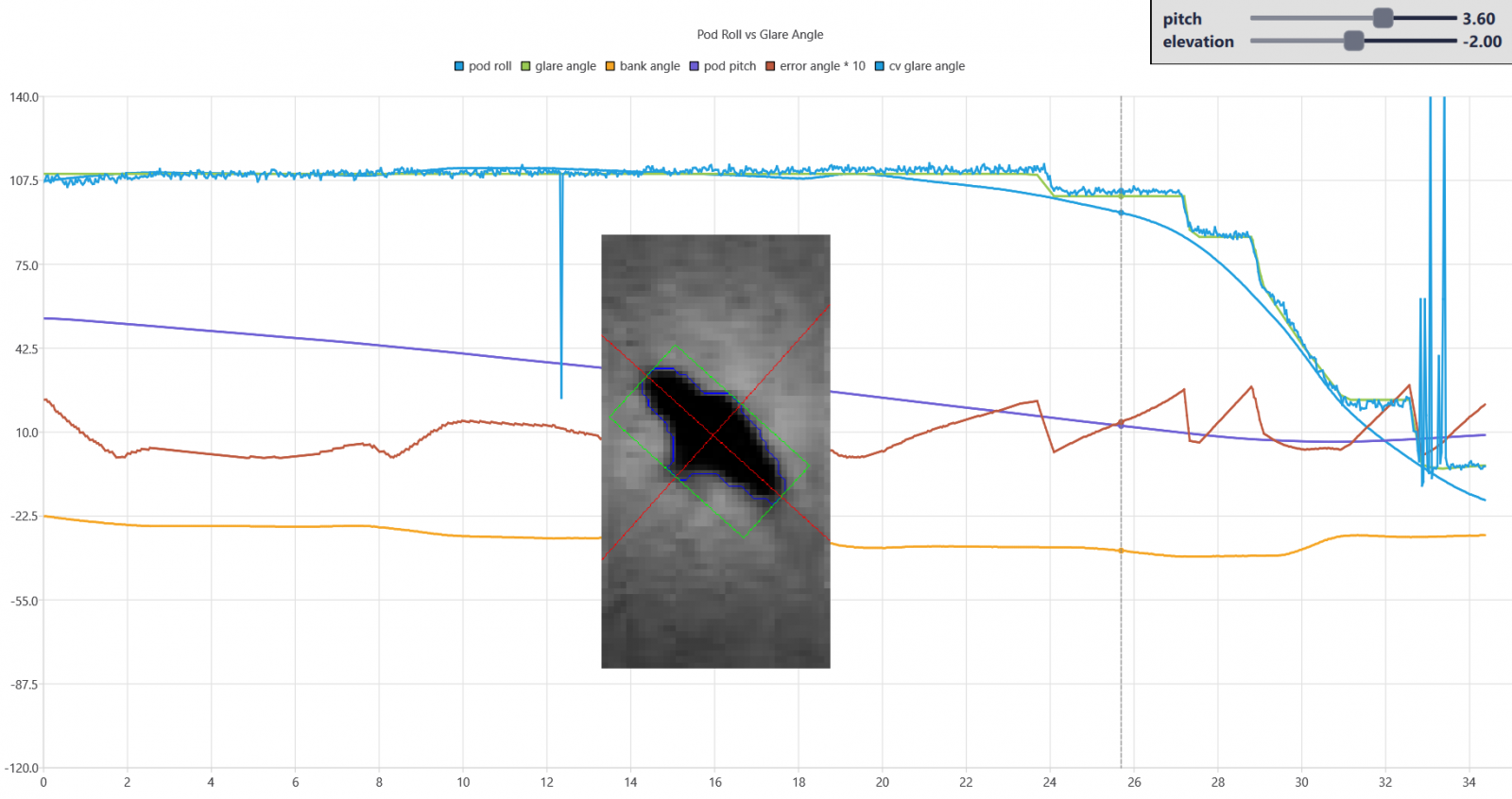Some have claimed that during the first 20 seconds of the video the object is fixed to the horizon/bank angle instead of the camera, but that is not what I found: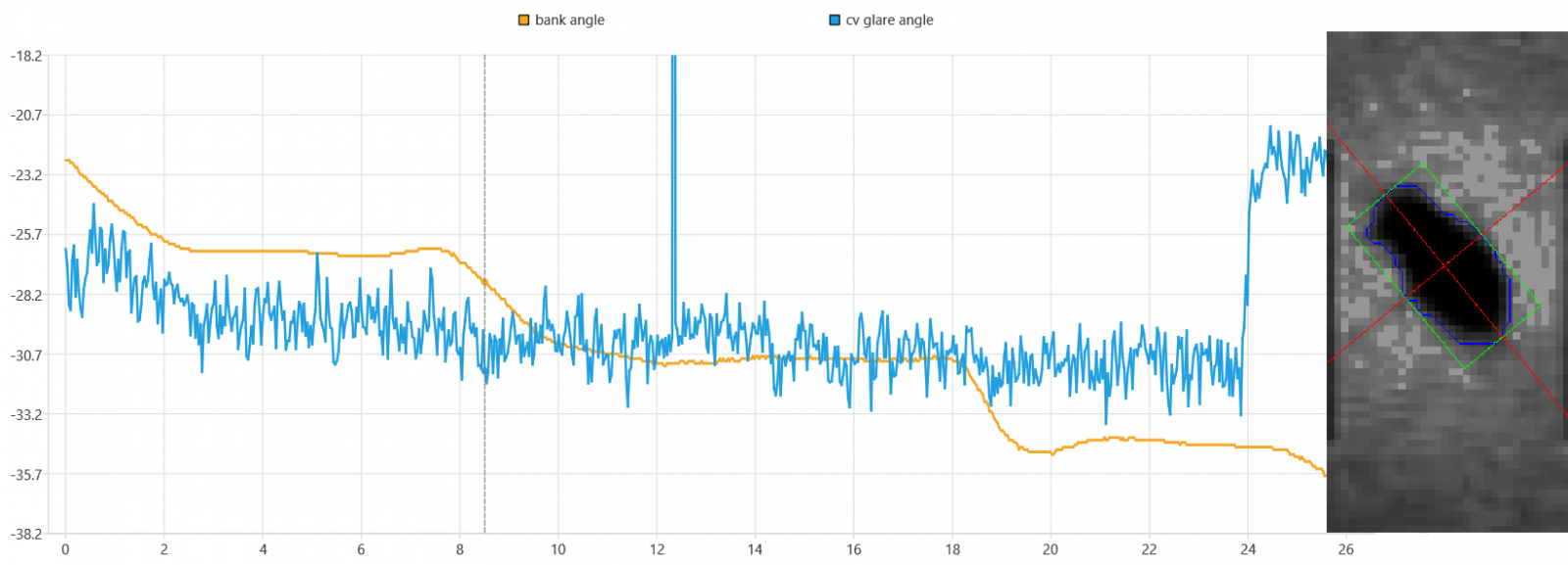There have been some questions about a bump during the first seconds of the video. I found that this bump was already discussed here and the long motion lines in this video can be seen bumping along with it as well, but I have some further observations.
In pretty much all of the unsmoothed measured angle data (not just mine but e.g the ones from the original gimbal sim too, shown below) I can see a small upward trend of a couple degrees during the first seconds. The deviation is small enough that just drawing a straight line through the first 20 seconds could still be correct, but maybe some rotation during the first seconds might be a slightly better fit.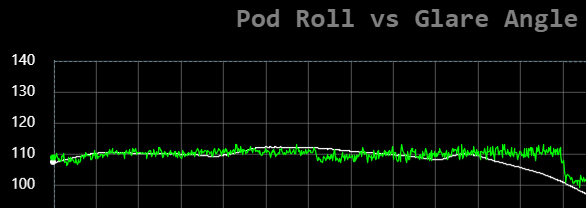Notice that the error angle at the beginning of the video is almost the same value as all the other peaks that coincide with the pod starting to rotate (by the way there's a small data error at 24 seconds, as the peaks are much more consistent without keyframing) but if the pod rotated during the first seconds then this should be reflected in the keyframed glare angles, which would change the values of the error angles.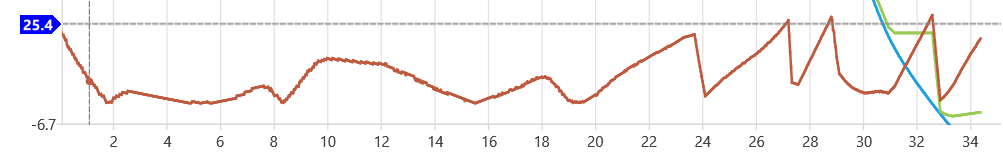I plotted the y coordinate of the center of mass of the object (centered to 0 and magnified 20 times). The large upward spikes outside of A-E are just a result of the algo not working for some frames there.If you look closely, B-E all start with a decrease of y coordinate (object moving up), followed by a large swing in the other direction. In D that second deviation is smaller. I think this fits with the idea that in B,C,E soon after the pod accelerates to start rotating it decelerates rapidly to stop again, while in D the deceleration is much smoother going into a continuous rotation phase. That could also explain why there's no noticeable bump near F, the deceleration being less abrupt. In A the y coordinate first increases (object goes down) and there's no noticeable oscillation after that. So maybe it's caused by a smaller change in pod rotation, in the opposite direction as B-E, with a soft tail edge. The variance of the y coordinate (over +/- 3 frames) at A is about 3x smaller than B-C, and those are changes of about 12 degrees, so let's try adding a proportionally smaller edge at A of about 4 degrees: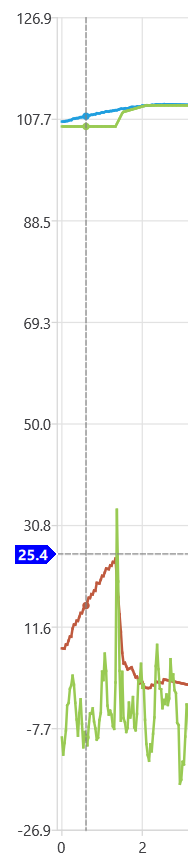The most striking feature here is that now the error angle happens to reach the same value as the other peaks did when the pod started rotating, at the right time to coincide with the bump. I didn't fine tune it to make it that way. This was the result of my first guess about the shape, size and location of that edge based on my reasoning outlined above. This to me is another strong indication of the predictive power of this model. With all these lines of evidence supporting it, I think we can say this small rotation likely did occur ?

Last edited:

Staff member

#### Fleck

##### New Member
Is there a reason that you're all using Euler angles instead of quarternion?

#### Mick West

Staff member
Is there a reason that you're all using Euler angles instead of quarternion?
Yes, two reasons. Firstly the view vector of the pod is given on-screen by azimuth and elevation, which are Euler angles. So we need to use those both as input and outputs. Secondly, a two-axis gimbal system is inherently limited to Euler angles, as it has a "pitch" and a "roll", and the limitation of using physical Euler angles is largely what creates the gimbal roll issue.

#### logicbear

##### New Member
not trying to predict the rotation steps
I wrote a very basic (incomplete) attempt to do that. I think the position of the first bump at the start of the video depends on what happens before the video starts, so I picked frame 111 as a starting point where the error in the previous error angle graph was 0. I go on from there keeping the roll constant until the error (which in this case does not depend on the glare angle anymore) goes above 2.5 degrees then I snap to the current ideal roll value and start a new step. I get the following graph: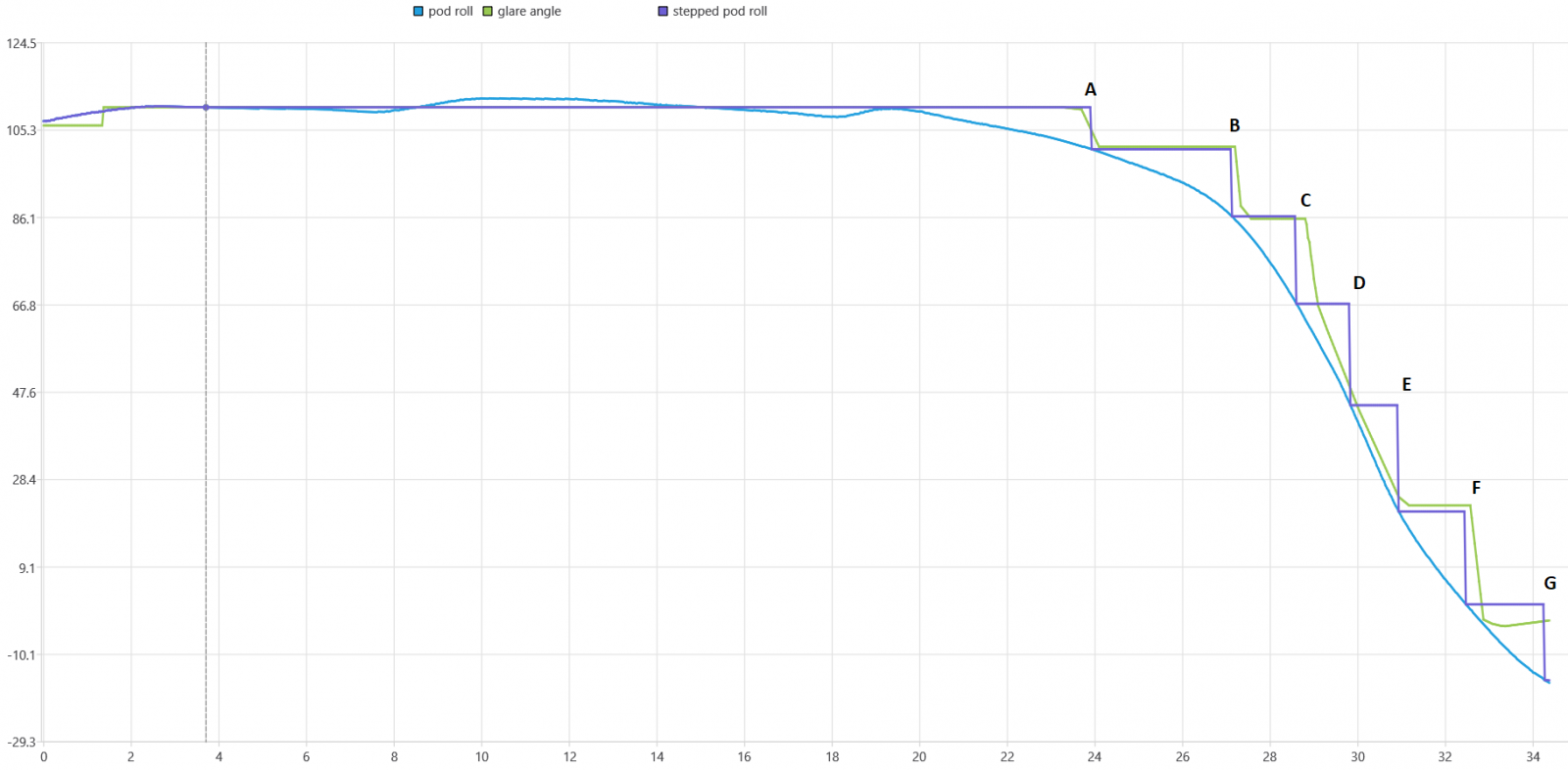For some time I had this idea that the stepped behavior is caused by going through az = 0 but actually that's exactly where the pod seems to choose *not* to use the stepped behavior. It could do the steps denoted by D, E, but it rotates smoothly instead. So the question is why would the pod do this ? Why does it stop using the stepped behavior after C, at around 2.6-3.3 degrees left ? Why does it start using the stepped pod behavior after E, at around 1 degree right ?

The patent says rolling the pod could be power consuming and not particularly agile or accurate, so if you can get away with using the internal mirrors for as long as possible, that sounds like a good idea. But the sudden acceleration of the pod seems to cause bumps and if that happens too often it seems like it could easily have an adverse effect on tracking, too much stress on the pod. So maybe the pod tries to make a prediction based on a bunch of factors, and only chooses the stepped behavior if it is likely to be able to keep using the same roll for at least 1.5 seconds ? Both of the shortest steps that the pod chose to do (C, F) seem to have this length. D and E would've only been around 1.2 seconds or less in length.

C++:
``````void calculate_stepped_pod_roll(std::vector<double>& stepped_pod_roll) {
stepped_pod_roll.resize(Frames);
int init_frame = 111;
for (int frame = 0; frame < Frames; frame++) {
auto [pitch, globalRoll] = pitchAndGlobalRollFromFrame(frame);
double ideal_podRoll = globalRoll - jetRollFromFrame(frame);
if (frame <= init_frame) {
stepped_pod_roll[frame] = ideal_podRoll;
} else {
vec3d stepped_v = PRJ2XYZ(pitch, stepped_pod_roll[frame - 1] + jetRollFromFrame(frame), jetPitchFromFrame(frame), 1);
vec3d v = PRJ2XYZ(pitch, globalRoll, jetPitchFromFrame(frame), 1);
double errorAngle = degrees(v.angleTo(stepped_v));
double error_threshold = 2.5;
if (errorAngle > error_threshold) {
stepped_pod_roll[frame] = ideal_podRoll;
} else {
stepped_pod_roll[frame] = stepped_pod_roll[frame - 1];
}
}
}
}``````

Last edited:

#### Mick West

Staff member
I get the following graph:If you ignore D and E, then that's crazy close! As I mention in my video, that portion (C-E) coincides with the jet banking, which introduced a new variable. Perhaps the algorithm takes some actual or predicted jet roll into account.

#### logicbear

##### New Member
that portion (C-E) coincides with the jet banking
It needs to take that into account for sure, but that banking only starts at around 1.1 degrees left, around D, and soon after it passes C, 2.6-3.3 degrees left, the pod already switches off the stepped behavior. That banking is in the opposite direction of every other change in bank angle during the video, and I assume caused by the pilot's decision at that time (D), not some autopilot, so there's probably no way the algorithm can predict it at C already. Or is the jet's autopilot more advanced than I'm assuming ?

Last edited:

#### dimebag2

##### Active Member
Perhaps the algorithm takes some actual or predicted jet roll into account.
Maybe a stupid question, but do you think the radar data influences the pod in any way? Could it be that the pod had this unusual behavior because of it was tracking an object with an unusual flight path. I'm thinking, if the FLIR "knows" the object is going slow or being stationary, maybe it's going in a "refinement mode" and it's using the internal gimbal axis to adapt to the target motion. Pure speculation here, but it could explain the puzzle and why both sides of the argument have valid points.

On that note, do we agree that :

- the gimbal includes 4 axis, 2 from the outer gimbal (pitch/roll), 2 from internal gimbal c-mirrors.

- according to this patent https://patents.google.com/patent/US9121758B2/en
the internal c-mirrors "may be of small angular travel (for example, less than or equal to 5 degrees). As a result, axis travels around the roll axis and avoids the gimbal singularity." In other words the internal roll axis (4th axis) should only be used inside ±3 degree of singularity to avoid gimbal lock

- there are additional internal mirrors that keep the object centered, but those have a range of only a few mradians (~tenth of degrees).

According to the patent, the internal 4th gimbal axis should not be used during the step rotation because they are happening outside the ±3 degree interval around singularity. And the internal mirrors cannot move for more than a few tenth of degrees, so they are not responsible for the 2.5degree tracking that is then recentered by rotation of the outer gimbal, according to the glare theory.

What is the explanation for the step rotation then, and based on what section of the patent exactly?

#### Mick West

Staff member
What is the explanation for the step rotation then, and based on what section of the patent exactly?
I think it's rather misleading to treat the patent as some kind of definitive description of what goes on in the ATFLIR. There's several patents, and they each describe embodiments of one aspect in a way that is meant to be a patent (for intellectual property protection), and not a helpful guide for reverse-engineers. They are also replete with examples, (like in your quote) and not solid figures. Sometimes there are different ways mentioned of doing things (like the dero being physical or digital).

The patent "Four-axis gimbaled airborne sensor having a second coelostat mirror to rotate about a third axis substantially perpendicular to both first and second axes" is really about THAT, and while it gives us clues as to what is going on in an ATFLIR, it's not a blueprint. The word "example" occurs 42 times, and "one embodiment" occurs 22 times!

See emphasised lines below:

Still other aspects, embodiments, and advantages of these exemplary aspects and embodiments are discussed in detail below. Embodiments disclosed herein may be combined with other embodiments in any manner consistent with at least one of the principles disclosed herein, and references to “an embodiment,” “some embodiments,” “an alternate embodiment,” “various embodiments,” “one embodiment” or the like are not necessarily mutually exclusive and are intended to indicate that a particular feature, structure, or characteristic described may be included in at least one embodiment. The appearances of such terms herein are not necessarily all referring to the same embodiment.
BRIEF DESCRIPTION OF THE DRAWINGS
Various aspects of at least one embodiment are discussed below with reference to the accompanying figures, which are not intended to be drawn to scale. The figures are included to provide illustration and a further understanding of the various aspects and embodiments, and are incorporated in and constitute a part of this specification, but are not intended as a definition of the limits of the invention.

Having described above several aspects of at least one embodiment, it is to be appreciated various alterations, modifications, and improvements will readily occur to those skilled in the art. Such alterations, modifications, and improvements are intended to be part of this disclosure and are intended to be within the scope of the invention. Accordingly, it is to be appreciated that embodiments of the methods and apparatuses discussed herein are not limited in application to the details of construction and the arrangement of components set forth in the above description or illustrated in the accompanying drawings. The methods and apparatuses are capable of implementation in other embodiments and of being practiced or of being carried out in various ways. Examples of specific implementations are provided herein for illustrative purposes only and are not intended to be limiting. Also, the phraseology and terminology used herein is for the purpose of description and should not be regarded as limiting. The use herein of “including,” “comprising,” “having,” “containing,” “involving,” and variations thereof is meant to encompass the items listed thereafter and equivalents thereof as well as additional items. References to “or” may be construed as inclusive so that any terms described using “or” may indicate any of a single, more than one, and all of the described terms. The scope of the invention should be determined from proper construction of the appended claims, and their equivalents.
Content from External Source

#### dimebag2

##### Active Member
So the hypothesis is that the Navy had their ATFLIR pods designed to roll in steps every 2.5deg motion of the internal gimbal axes. Not only at singularity, but every time pod roll is needed. I'd be curious to see a faster-than-Gimbal moving target crossing the FOV. This must induce bumps and loss of lock all along. Maybe they don't want these videos to be classified because it shows how weak their pods are.

What about this? It's along the lines of the algorithm predicting jet roll, this can only be done if information on the target trajectory is fed to the ATFLIR, no?

Do you think the radar data influences the pod in any way? Could it be that the pod had this unusual behavior because of it was tracking an object with an unusual flight path. I'm thinking, if the FLIR "knows" the object is going slow or being stationary, maybe it's going in a "refinement mode" and it's using the internal gimbal axis to adapt to the target motion. Pure speculation here, but it could explain the puzzle and why both sides of the argument have valid points.

#### Mick West

Staff member
So the hypothesis is that the Navy had their ATFLIR pods designed to roll in steps every 2.5deg motion of the internal gimbal axes. Not only at singularity, but every time pod roll is needed.
No, the hypothesis is broadly that if the error is under 2.5 degrees (for example, but that sure seems to work here), then it relies on the internal mirrors. The precise algorithm is unknown.

The error is the angle between where the pod head is physically pointing, ignoring the mirrors (green dot), and where the target is (white dot)

#### dimebag2

##### Active Member
Yes but a larger error has to pass by 2.5deg, so the algorithm you suggest would activate pod roll every time the errors goes beyond 2.5deg (making faster steps). Unless it considers how fast the deviation goes above 2.5deg, and activate pod roll when the internal system can't catch up. Unfortunately we can only speculate on how the pod exactly works. Crazy that nobody from Raytheon or the Navy can settle this question for good, it would be about time.

Too bad you don't reply to my other point. But that's fine.

#### logicbear

##### New Member
every time pod roll is needed. I'd be curious to see a faster-than-Gimbal moving target crossing the FOV. This must induce bumps and loss of lock all along.
.... this can only be done if information on the target trajectory is fed to the ATFLIR, no?
No, doing it every time pod roll is needed would be inconsistent with the video (D,E). A possibility that I suggested was that it "only chooses the stepped behavior if it is likely to be able to keep using the same roll for at least 1.5 seconds" . The faster the target is moving the shorter those steps near az = 0 would become. So IF the algorithm is doing what I suggested then you'd expect the pod to use the stepped behavior less for faster targets. The relevant speed here is the target's angular velocity, and the pod could estimate that information from tracking the object visually so I don't think it would need more information about the target trajectory. Optionally as an improvement it could use any information the ownship's autopilot can give it about future expected changes in the jet's own pitch/yaw/bank angles.

#### Mick West

Staff member
Maybe a stupid question, but do you think the radar data influences the pod in any way? Could it be that the pod had this unusual behavior because of it was tracking an object with an unusual flight path. I'm thinking, if the FLIR "knows" the object is going slow or being stationary, maybe it's going in a "refinement mode" and it's using the internal gimbal axis to adapt to the target motion. Pure speculation here, but it could explain the puzzle and why both sides of the argument have valid points.
What the object is doing isn't exactly relevant to the pod, it's what that ends up in terms of az/el changes when combined with the jet motion. Since the Az/El motion is exactly the same for the near of far paths (or any LOS traversal) then I don't know how you could adapt to one particular motion. It would see that it would be LESS likely to do something if the motion was highly unusual unless the algorithm is something like "is it's moving unpredictably then go forward in steps as it might reverse direction at any second" But that seems like an unlikely situation they would program for.

#### Mendel

##### Senior Member.
do we know if the pod can roll at different speeds?